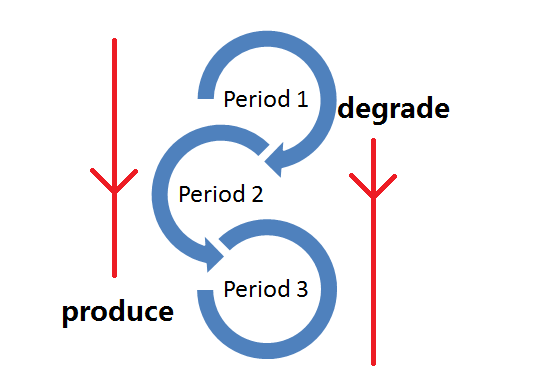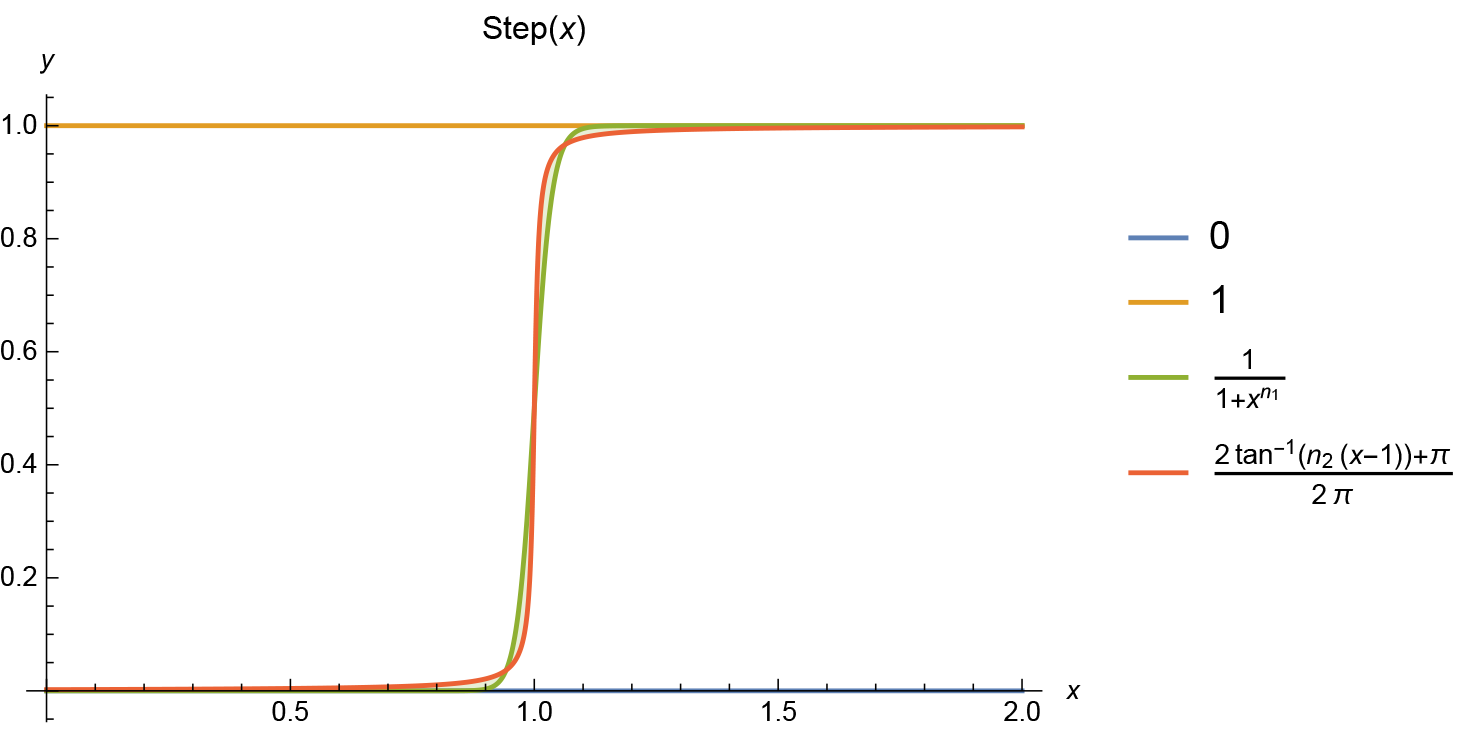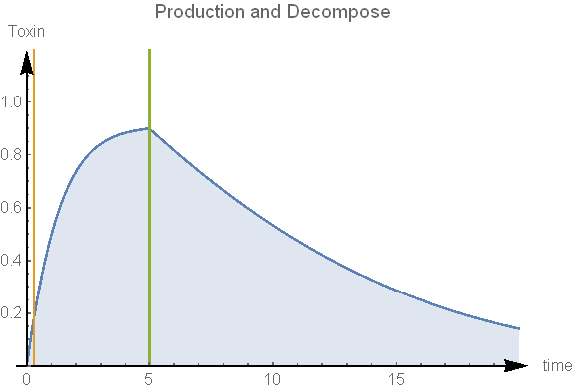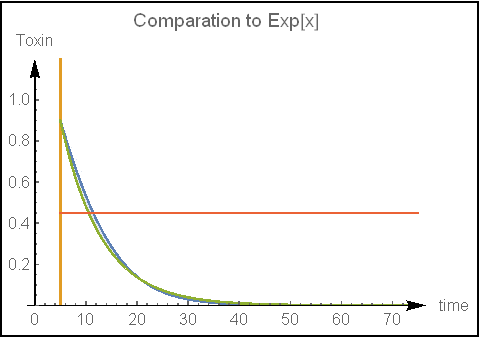# Team:ZJU-China/Modeling/third

## Abstract

Toxalbumin is produced by cell, and resolved by enzyme or itself.We built model for the process basic on Michaelis-Menten equation.

### Keywords

Michaelis-Menten equation

Mass prediction

## Introduction

There are four important parameters in our design, which are $${CNC-digest-Time}$$, $${CNC-degradation-Time}$$, $${Toxin-CNC-Time}$$ and $${Toxin-air-Time}$$. Respectively, $${CNC-digest-Time}$$ is the time when the bait comes into another termite's gut(trophallaxis) and the CNC-coat is completely digest there. $${CNC-degradation-Time}$$ is when CNC-coat degrades in national condition. It should be larger than $${CNC-digest-Time}$$ according to our general knowledge. $${Toxin-CNC-Time}$$ is the degradation time of Toxin in CNC-coat while $${Toxin-air-Time}$$ is that in the air.

Ideally, the relationship of these four parameters is as followed.

$${CNC-digest-Time} \le {Toxin-CNC-Time} \le {CNC-degradation-Time}$$

$$or: {Toxin-air-Time} \ll {CNC-degradation-Time}$$

The first inequation ensure that Toxin will not lost its effect so quickly or slowly. It is the termite that we try to kill. While the next one, means even though the CNC-coat is degraded, Toxin will degrade right away so that it will not cause environment pollution.

In this page, we model the process of the production and degrade of Toxin to find the influence to $${Toxin-CNC-Time}$$, or the degradation time of Toxin in CNC-coat.

## Michaelis-Menten equation

### Assumptions

Michaelis-Menten equation is the velocity equation of the relationship between the initial velocity of the enzymatic reaction and the concentration of substrate is indicated. We suppose the producing and degradation observe this law.

We divided the whole process in to three period. During the first two period, cell produce Toxin. In the last two period, Toxin starts to degrade.### Producing Equation and Degrading Equation

In the producing equation, Toxin is the production.

$$v_{Toxin}=\frac{V_{max}[S]}{K_m+[S]}$$

Here $$v_{Toxin}$$ is the velocity that Toxin is produced. $$V_{max}$$ is the producing velocity when substrate is saturated. $$[S]$$ is substrate's concentration. And $$K_m$$ is called Michaelis constant.

In the producing equation, Toxin is the substrate.

$$v_{Toxin}=-\frac{V_{max,2}[{Toxin}]}{K_{m,2}+[{Toxin}]}$$

$$V_{max,2}$$ is the reducing velocity when Toxin is saturation, and $$K_{m,2}$$ is another Michaelis constant

The two equation seems simple in separate formate, but it became complex when considering time factor. We will discuss is in the next section.

To build the function into ordinary function, the relationship is added.

$$v_{Toxin}=\frac{\partial [{Toxin}]}{\partial t}$$

### Time Factor

We do not have enough time to measure the exact time when termite digest, but I suppose we have got it, as well as the time that Toxin starts to resolve. They are $$t_{2}$$ and $$t_1$$, respectively.

If time is less than $$t_1$$, the function is:

$$\frac{\partial [{Toxin}]}{\partial t} = \frac{V_{max}[S]}{K_m+[S]}$$

If time is greater than $$t_1$$ but less than $$t_2$$:

$$\frac{\partial [{Toxin}]}{\partial t} = \frac{V_{max}[S]}{K_m+[S]} - \frac{V_{max,2}[{Toxin}]}{K_{m,2}+[{Toxin}]}$$

If greater:

$$\frac{\partial [{Toxin}]}{\partial t} = - \frac{V_{max,2}[{Toxin}]}{K_{m,2}+[{Toxin}]}$$

Still, use function $$\arctan$$ take the place of comparing, we get the final function. In the ordinary differential function, we can solve the two condition respectively, but a continues function will enhance the flexibility and simplicity. Still, use function $$\arctan$$ take the place of comparing, we get the final function, for the simple reason that its infinite order is derivable, and can be regarded as step function easily.

$$\begin{eqnarray*} \frac{\partial [{Toxin}]}{\partial t} &=&(1-step(t,t2)) \frac{V_{max}[S]}{K_m+[S]} - step(t,t1) \frac{V_{max,2}[{Toxin}]}{K_{m,2}+[{Toxin}]}\\ step(x,x0)&=&\frac{2\arctan(100(x-x0))+\pi}{2\pi} \end{eqnarray*}$$

$$step$$ function can be placed by $$\frac{1}{{x^{-100}}+1}$$ etc. We compare the two function here. By adjusting the parameters $$n_1,n_2$$, both function approached well. Because $$n_2$$ must be even and $$\frac{1}{{x^{-100}}+1}$$ is the integral, we choose the last one.## Solution

With some typical parameter, we use NDSolve function to solve the ordinary differential equation, the answer is as below. For the time $$t_1$$ is much less than $$t_2$$, the change at that time is not obvious. When the producing reaction stop, the concentration starts to drop.## Application

In this model, we care more about safty.

What we concern is that when our CNC-coat is degraded, the Toxin is still in the cell. Although we can premise that the Toxin will be separate by CNC-coat, and our device, as a second protection, can stop Toxin's spread, the only device's protection may not be really enough. Wind or other flowing air will cause the spread. An effective way is to measure the Toxin's half-life period.To study the tendency of the reaction, the figure is at a long time (three of four times than $$t_2$$), and the dark green curve is a standard $$exp$$ function curve. Approximately, we can regard the curve as the rad line. Measuring the half-life period, the model shows that the deviation is under 20% or so. We will know the allowance of Toxin easily.

introduction